# Fraction calculator

The calculator performs basic and advanced operations with fractions, expressions with fractions combined with integers, decimals, and mixed numbers. It also shows detailed step-by-step information about the fraction calculation procedure. Solve problems with two, three, or more fractions and numbers in one expression.

## Result:

### 3/5 * 3/7 = 9/35 ≅ 0.2571429

Spelled result in words is nine thirty-fifths.

### How do you solve fractions step by step?

1. Multiple: 3/5 * 3/7 = 3 · 3/5 · 7 = 9/35
Multiply both numerators and denominators. Result fraction keep to lowest possible denominator GCD(9, 35) = 1. In the next intermediate step, the fraction result cannot be further simplified by canceling.
In words - three fifths multiplied by three sevenths = nine thirty-fifths.

#### Rules for expressions with fractions:

Fractions - use the slash “/” between the numerator and denominator, i.e., for five-hundredths, enter 5/100. If you are using mixed numbers, be sure to leave a single space between the whole and fraction part.
The slash separates the numerator (number above a fraction line) and denominator (number below).

Mixed numerals (mixed fractions or mixed numbers) write as non-zero integer separated by one space and fraction i.e., 1 2/3 (having the same sign). An example of a negative mixed fraction: -5 1/2.
Because slash is both signs for fraction line and division, we recommended use colon (:) as the operator of division fractions i.e., 1/2 : 3.

Decimals (decimal numbers) enter with a decimal point . and they are automatically converted to fractions - i.e. 1.45.

The colon : and slash / is the symbol of division. Can be used to divide mixed numbers 1 2/3 : 4 3/8 or can be used for write complex fractions i.e. 1/2 : 1/3.
An asterisk * or × is the symbol for multiplication.
Plus + is addition, minus sign - is subtraction and ()[] is mathematical parentheses.
The exponentiation/power symbol is ^ - for example: (7/8-4/5)^2 = (7/8-4/5)2

#### Examples:

subtracting fractions: 2/3 - 1/2
multiplying fractions: 7/8 * 3/9
dividing Fractions: 1/2 : 3/4
exponentiation of fraction: 3/5^3
fractional exponents: 16 ^ 1/2
adding fractions and mixed numbers: 8/5 + 6 2/7
dividing integer and fraction: 5 ÷ 1/2
complex fractions: 5/8 : 2 2/3
decimal to fraction: 0.625
Fraction to Decimal: 1/4
Fraction to Percent: 1/8 %
comparing fractions: 1/4 2/3
multiplying a fraction by a whole number: 6 * 3/4
square root of a fraction: sqrt(1/16)
reducing or simplifying the fraction (simplification) - dividing the numerator and denominator of a fraction by the same non-zero number - equivalent fraction: 4/22
expression with brackets: 1/3 * (1/2 - 3 3/8)
compound fraction: 3/4 of 5/7
fractions multiple: 2/3 of 3/5
divide to find the quotient: 3/5 ÷ 2/3

The calculator follows well-known rules for order of operations. The most common mnemonics for remembering this order of operations are:
PEMDAS - Parentheses, Exponents, Multiplication, Division, Addition, Subtraction.
BEDMAS - Brackets, Exponents, Division, Multiplication, Addition, Subtraction
BODMAS - Brackets, Of or Order, Division, Multiplication, Addition, Subtraction.
GEMDAS - Grouping Symbols - brackets (){}, Exponents, Multiplication, Division, Addition, Subtraction.
Be careful, always do multiplication and division before addition and subtraction. Some operators (+ and -) and (* and /) has the same priority and then must evaluate from left to right.

## Fractions in word problems:

• Lowest terms 2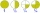The expression 4/12 can be expressed in its lowest term as 1/3. What is 3/15 expressed in its lowest term?
• ZdeněkZdeněk picked up 15 l of water from a 100-liter full-water barrel. Write a fraction of what part of Zdeněk's water he picked.
• Report 2A School reports students to teacher ratio of 6:1. If there are 45 teachers in the School, how many students are there?
• NumberI think the number. If I add to its third seven I get same as when to its quarter add 8. Which is the number?
• A marketA market vendor was able to sell all the mangoes, papayas, and star apples.  1/5  of the fruits were mangoes,   2/3  of them were papayas and the rest were star apples.  How many parts of the fruits sold are star apples?
• Golf ballsOf the 28 golf balls, 1/7 are yellow. How many golf balls are yellow? Use the model to help you. Enter your answer in the box.
• Chester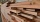Chester has a piece of wood measuring 1 2/3 cm. He needs to cut it to pieces measuring 3/4 cm long. How many pieces of wood did Chester cut?
• DIY press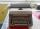Under socialism regime was in some socialist countries to own a typewriter requires special permission. That has hindered the spread of DIY literature (manually transcribed through carbon copy paper for typewriters). Calculate how many typewriters today c
• The rope 2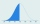The rope in the school gym is 10 ½ feet long. To make it easier to climb, Coach Hill tied a knot in the rope every ¾ foot. How many knots are in the rope?
• Seed germination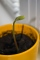Seed germination is 80%. 8% of the germinated plants subsequently die. How many seeds does a gardener have to sow in order to grow 2,000 plants?Skilled workshop master washes client car 1/5 hour, cleaned the client's car in 5/4 hour, and painted small defects on car 1 1/3 hour. How long did it take him to do all the necessary work tasks?Four pupils divided \$ 1485 so that the second received 50% less than the first, the third 1/2 less than a fourth, and fourth \$ 154 less than the first. How much money had each of them?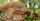Grandfather gathered fresh mushrooms. The fifth was wormwood, and we threw it away. The other dried up. He obtained 720 grams of dried mushrooms. How many kilograms did the grandfather collect, and by drying the mushrooms, they lost 75% of their weight?